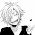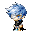## Fili's Rate Problems

Unit Rate -> Rate Problems
1. If Bob has 20 apples per one milk, how much milk would Bob have if he had 40 apples? (Group)2. Grandma's house is 500km away. Jill drove 50km per hour to grandma's house and it took 10 hours to get there, how many hours would it take if he drove 250km per hour? (My own)

Rates->Unit Rates Problem
1. If Jack has 10 chairs per 2 tables, how many chairs would one table have? (My Own)

2.If Julian has 10 snacks per 10 drinks, how many snacks would go with one drink? (My Own)

This is a video on how to solve rate problems using proportion
5:07 PM

#### 4 comments :

1.Fili your Group Unit Rate to Rate Problem's answer is 2 because i think an easier way is, if you double the apple that mean you double the milk. >.<

2.Ops, sorry Fili forgot my problem, so here it is:
If Anna has 40 apples per 2 milk, how much milk would Anna have if she had 80 apples?
>.<

3.Jack's Chair Problem
2 divided by 2 is 1 and 10 divided by 2 is 5 so that means its 5 chairs per table

If Jack has 32 chairs for every 4 tables. How many chairs would 16 tables have?

4.For Kallyl's Question: I have an answer of 4 milk because if you have 40 apples and x 2 its 80 apples so whatever you do on one side you do on the other so 2 x 2 is four So for every 80 apples, there's 4 milk.

For Zack's Question: I have an answer of 128 chairs because I multiplied 4 x 1 which is 1 and I multiplied 32 x 16 which gave me 512, then I 512/4 which game me 128. So, there's 128 chairs for 16 tables.